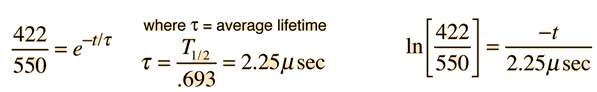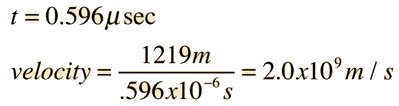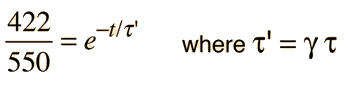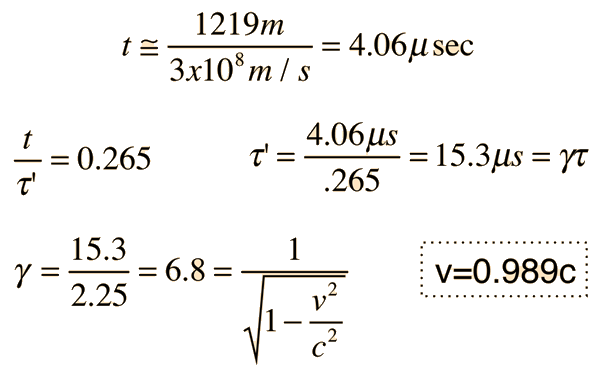# Muon Velocity

On top of a mountain at 6000 ft a muon detector measures a flux of 550 muons per hour. At a laboratory at the base of the mountain at 2000 ft, a simultaneous experiment measures 422 muons/hr. The half-life of the muon is 1.56 microseconds. How fast were the muons traveling?

Solution: First we will proceed without regard to relativity and the Lorentz transformation.

The distance traveled L=4000 ft x 0.3048 m/ft = 1219 m

The time is then calculated for the two populations as an exponential decay process using the half-life measured in the laboratory.The results of this calculation are:But this can't be right! This is 6.7 times the speed of light!

Having run into a serious violation of the speed limit of the universe on the first try, we shall attempt to include relativity in the calculation.

Considering time dilation, we must take into account that the average lifetime must be transformed by the Lorentz transformation.The problem with this is that there are two unknowns: we know neither the time nor the average lifetime. However, we can get a reasonable estimate of the average lifetime by assuming that the velocity is essentially =c. We use this assumption to calculate the time of transit.Length contraction and time dilation in the muon experiment
Index
HyperPhysics***** Relativity Go Back

# Some History of the Muon Experiment

The historical experiment upon which the model muon experiment is based was performed by Rossi and Hall in 1941. They measured the flux of muons at a location on Mt Washington in New Hampshire at about 2000 m altitude and also at the base of the mountain. They found the ratio of the muon flux was 1.4, whereas the ratio should have been about 22 even if the muons were traveling at the speed of light, using the muon half-life of 1.56 microseconds. When the time dilation relationship was applied, the result could be explained if the muons were traveling at 0.994 c.

In an experiment at CERN by Bailey et al., muons of velocity 0.9994c were found to have a lifetime 29.3 times the laboratory lifetime.

Index

References
Rossi, et al.

Bailey, et al.
HyperPhysics***** Relativity Go Back